Courses

# Test: Theory Of Cost- 3

## 30 Questions MCQ Test Business Economics for CA Foundation | Test: Theory Of Cost- 3

Description
This mock test of Test: Theory Of Cost- 3 for CA Foundation helps you for every CA Foundation entrance exam. This contains 30 Multiple Choice Questions for CA Foundation Test: Theory Of Cost- 3 (mcq) to study with solutions a complete question bank. The solved questions answers in this Test: Theory Of Cost- 3 quiz give you a good mix of easy questions and tough questions. CA Foundation students definitely take this Test: Theory Of Cost- 3 exercise for a better result in the exam. You can find other Test: Theory Of Cost- 3 extra questions, long questions & short questions for CA Foundation on EduRev as well by searching above.
QUESTION: 1

Solution:
QUESTION: 2

Solution:
QUESTION: 3

### A firm will close down in the short period if its average revenue is less than its:

Solution:
QUESTION: 4

The average fixed cost for producing an output of 6 units of a product by a firm is Rs. 30. The same cost for producing an output of 4 units will be Rs. _________.

Solution:
QUESTION: 5

Long run price is also called by the name of ________.

Solution:
QUESTION: 6

Which of the following cost curve will slope downward and does not touch the x-axis?

Solution:
QUESTION: 7

Consider the following data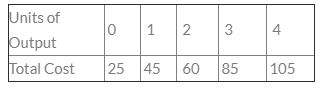The Average Variable Cost (AVC) for an output of 4 units will be:-

Solution:
QUESTION: 8

Average Revenue Curve is also known as _________.

Solution:
QUESTION: 9

Payment made to outsiders for their goods and services are called :

Solution:
QUESTION: 10

When AC curve is rising, the MC curve must be ________ to it

Solution:
QUESTION: 11

What will be the total fixed cost for the production of three units as per the details given below?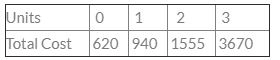Solution:
QUESTION: 12

A firm encountering economies of scale over some range of output will have a:

Solution:
QUESTION: 13

In which of the following cases opportunity cost concept applies?

Solution:
QUESTION: 14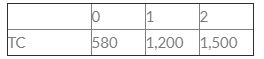Calculate AFC at 2nd unit of output

Solution:
QUESTION: 15

Which statement among below is correct in reference in Average Fixed Cost

Solution:
QUESTION: 16

Opportunity cost is :

Solution:
QUESTION: 17

What will be the TVC if we produce 2 units?
Units  0    1    2
TC    20  37  50

Solution:
QUESTION: 18

Which curve is never U-shaped?

Solution:
QUESTION: 19

External economies accrue due to ________:

Solution:
QUESTION: 20

Average Revenue Curve is also known as _________.

Solution:
QUESTION: 21

Which one of the following is correct?

Solution:
QUESTION: 22

When shape of average cost curve is upward, marginal cost :

Solution:
QUESTION: 23

Which of the following is known as Envelope curve?

Solution:
QUESTION: 24

Payment made to outsiders for their goods and services are called :

Solution:
QUESTION: 25

Long run price is also called by the name of ________.

Solution:
QUESTION: 26

AFC curve is:

Solution:

The average fixed costs AFC curve is downward sloping because fixed costs are distributed over a larger volume when the quantity produced increases. AFC is equal to the vertical difference between ATC and AVC. Variable returns to scale explains why the other cost curves are U-shaped.

QUESTION: 27

A firm’s average fixed cost is Rs. 40 at 12 units. What will be the average fixed cost at 8 units:

Solution:
QUESTION: 28

Which of the following cost curves is never ‘U’ shaped?

Solution:
QUESTION: 29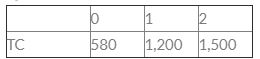Calculate AFC at 2nd unit of output

Solution:
QUESTION: 30

Calculate total cost of 4 units: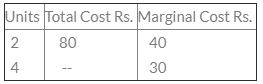Solution: